Ex 1.3

Chapter 1 Class 7 Integers
Serial order wise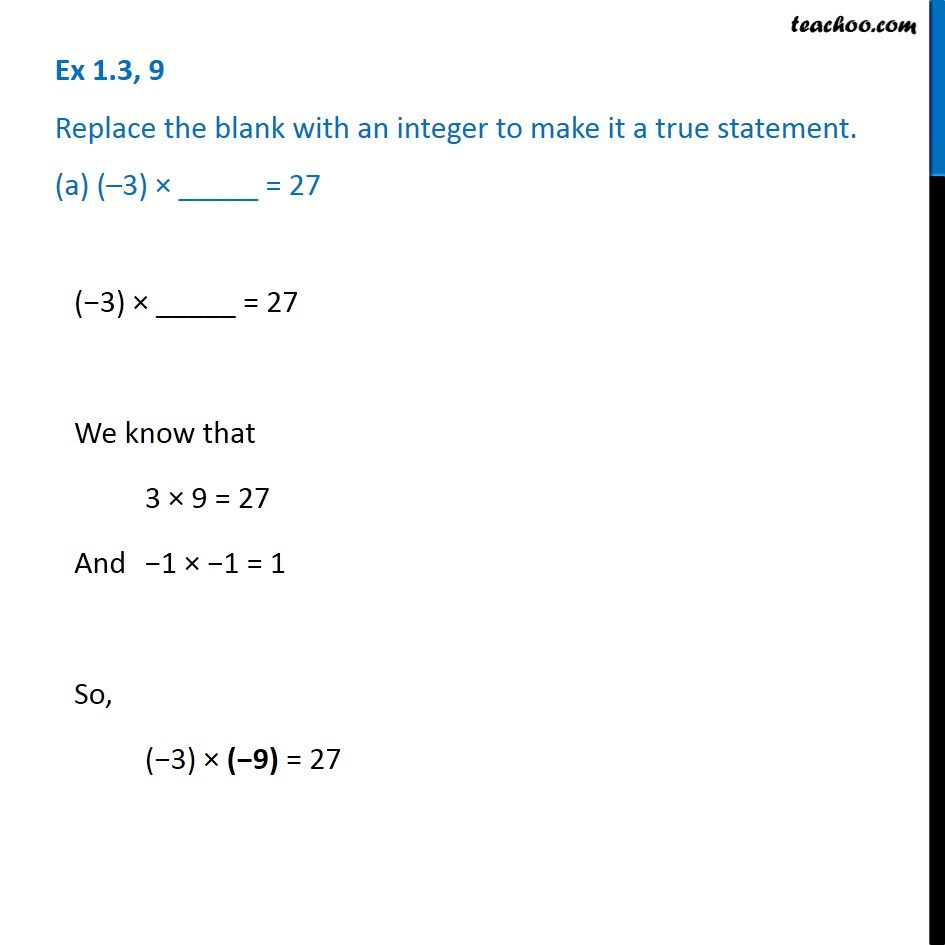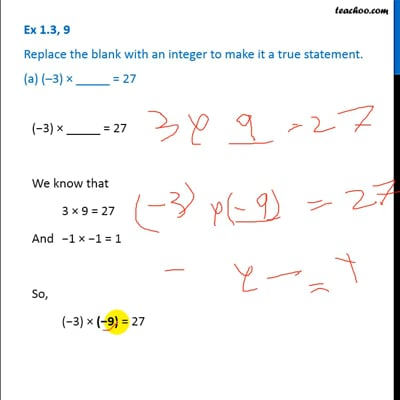This video is only available for Teachoo black users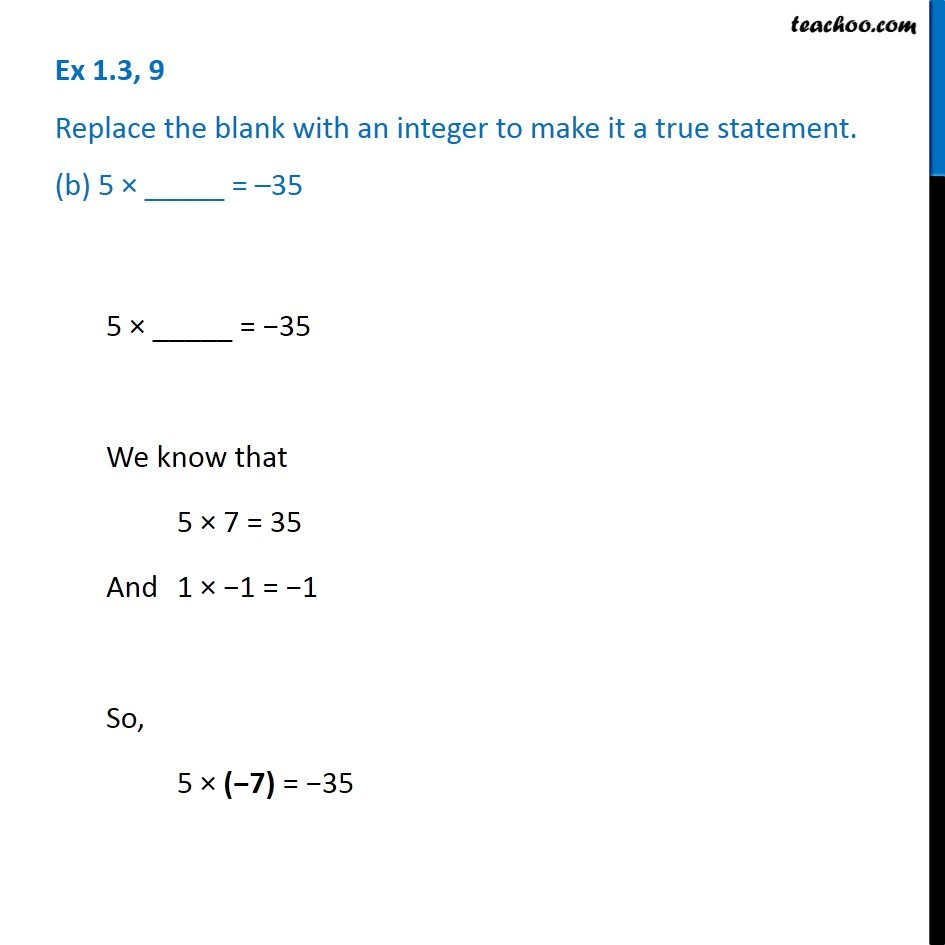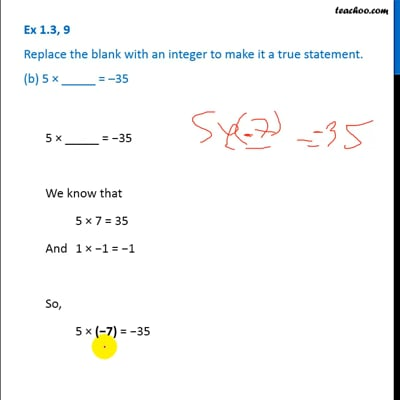This video is only available for Teachoo black users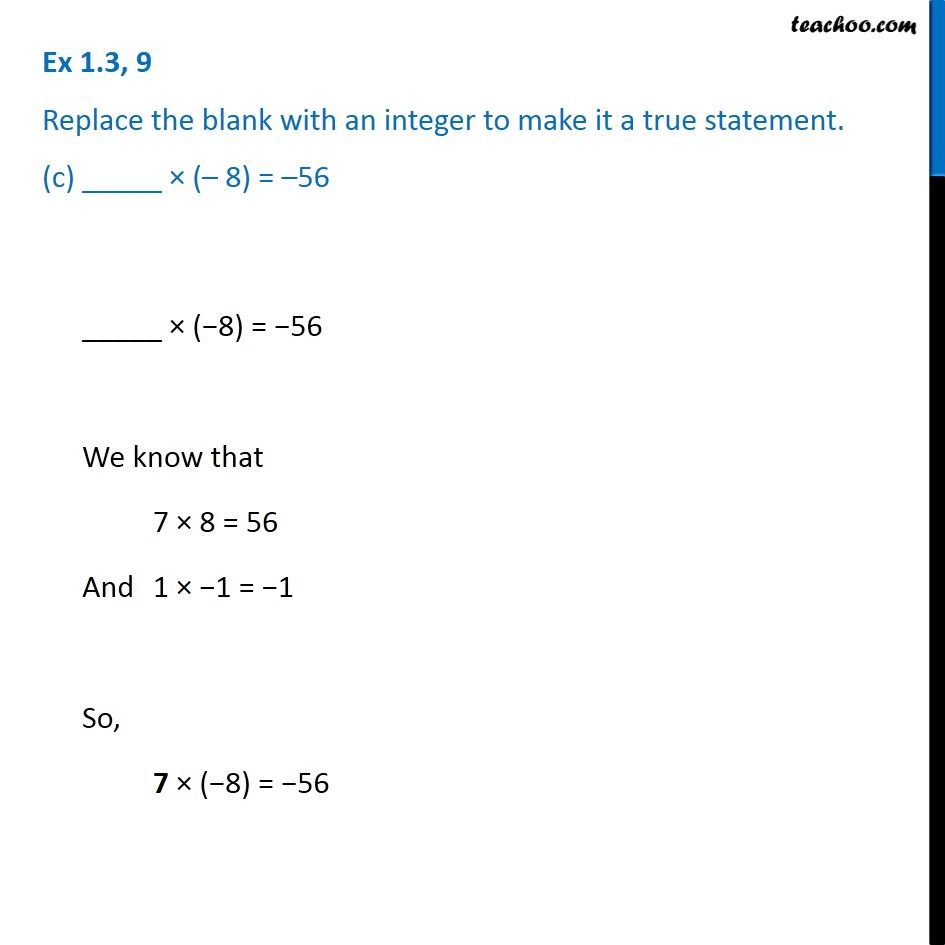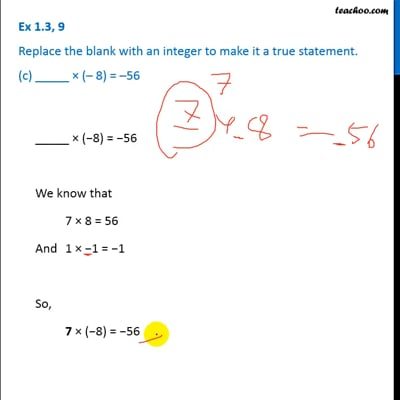This video is only available for Teachoo black users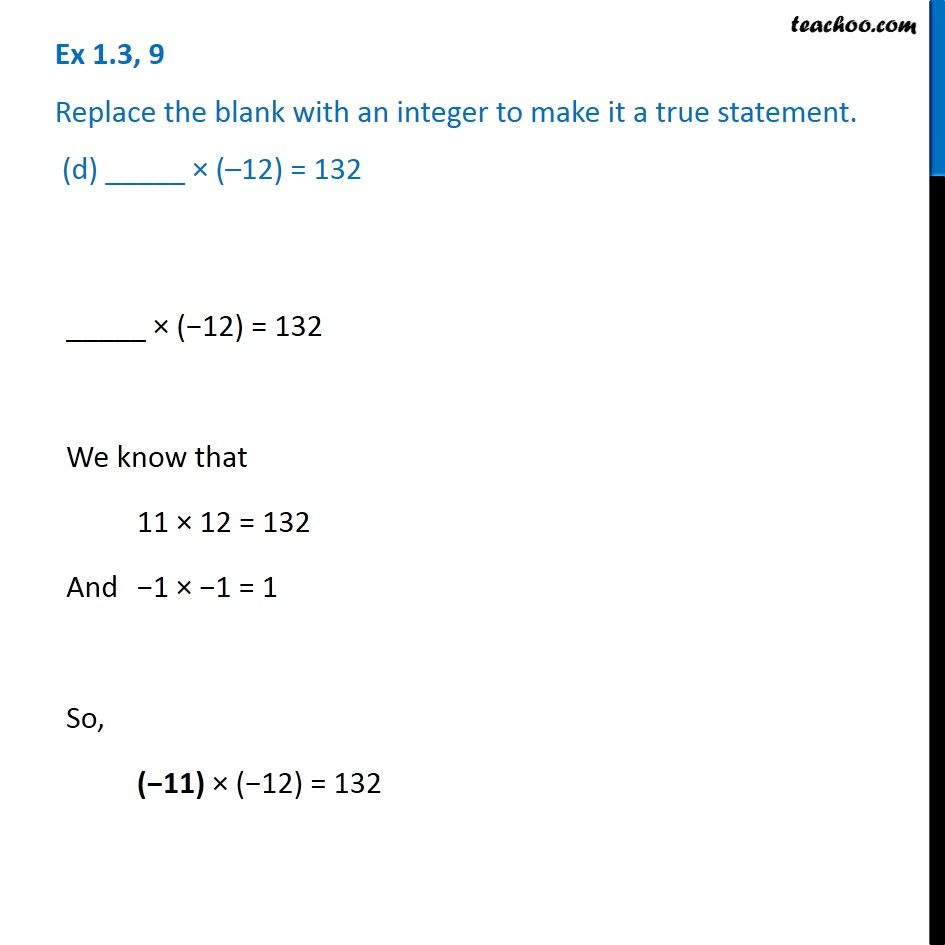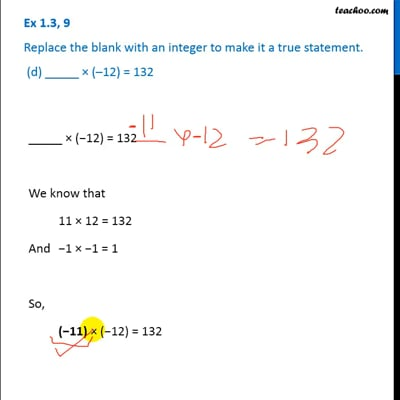This video is only available for Teachoo black users

### Transcript

Ex 1.3, 9 Replace the blank with an integer to make it a true statement. (a) (–3) × _____ = 27 (−3) × _____ = 27 We know that 3 × 9 = 27 And −1 × −1 = 1 So, (−3) × (−9) = 27 Ex 1.3, 9 Replace the blank with an integer to make it a true statement. (b) 5 × _____ = –35 5 × _____ = −35 We know that 5 × 7 = 35 And 1 × −1 = −1 So, 5 × (−7) = −35 Ex 1.3, 9 Replace the blank with an integer to make it a true statement. (c) _____ × (– 8) = –56 _____ × (−8) = −56 We know that 7 × 8 = 56 And 1 × −1 = −1 So, 7 × (−8) = −56 Ex 1.3, 9 Replace the blank with an integer to make it a true statement. (d) _____ × (–12) = 132 _____ × (−12) = 132 We know that 11 × 12 = 132 And −1 × −1 = 1 So, (−11) × (−12) = 132